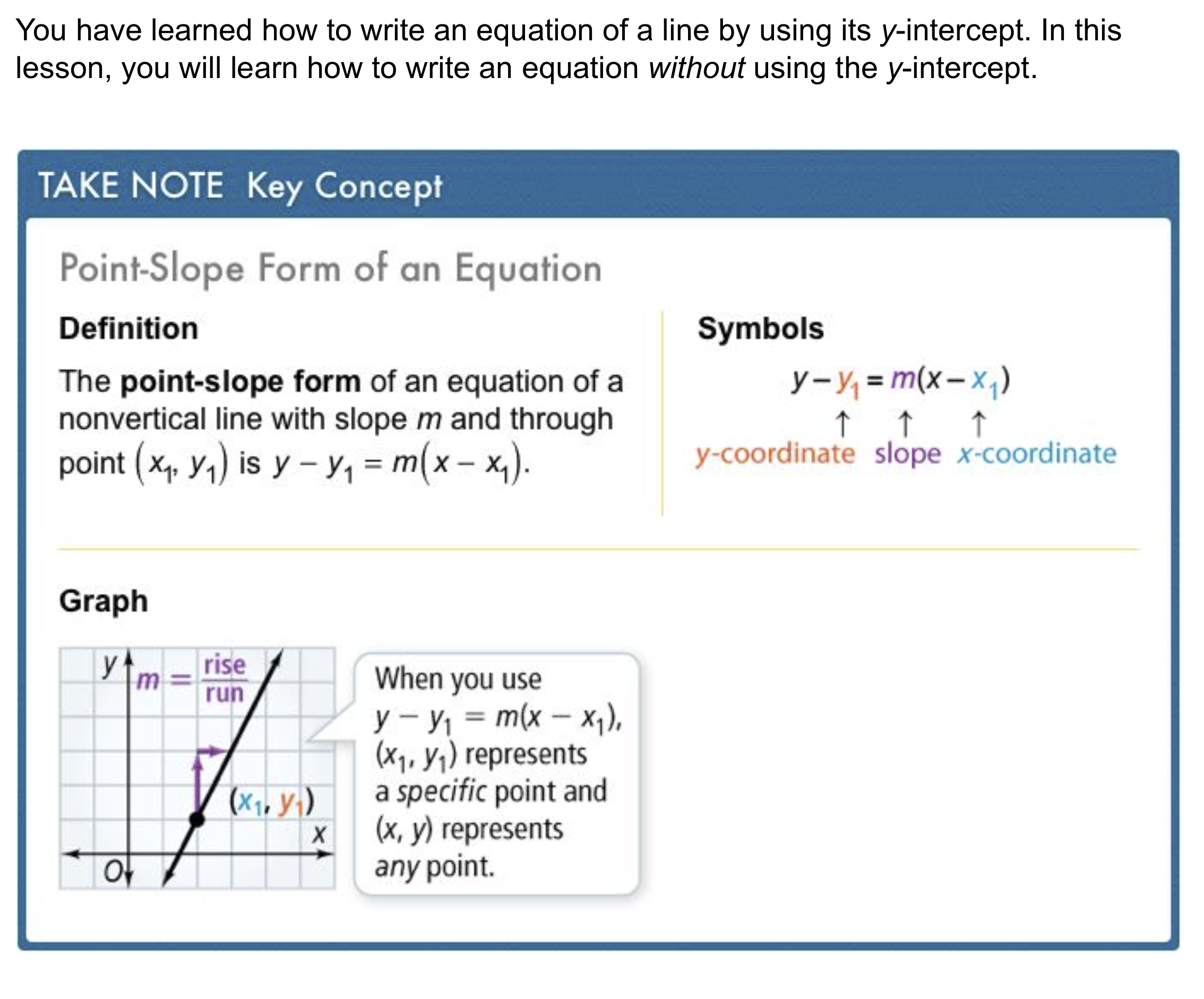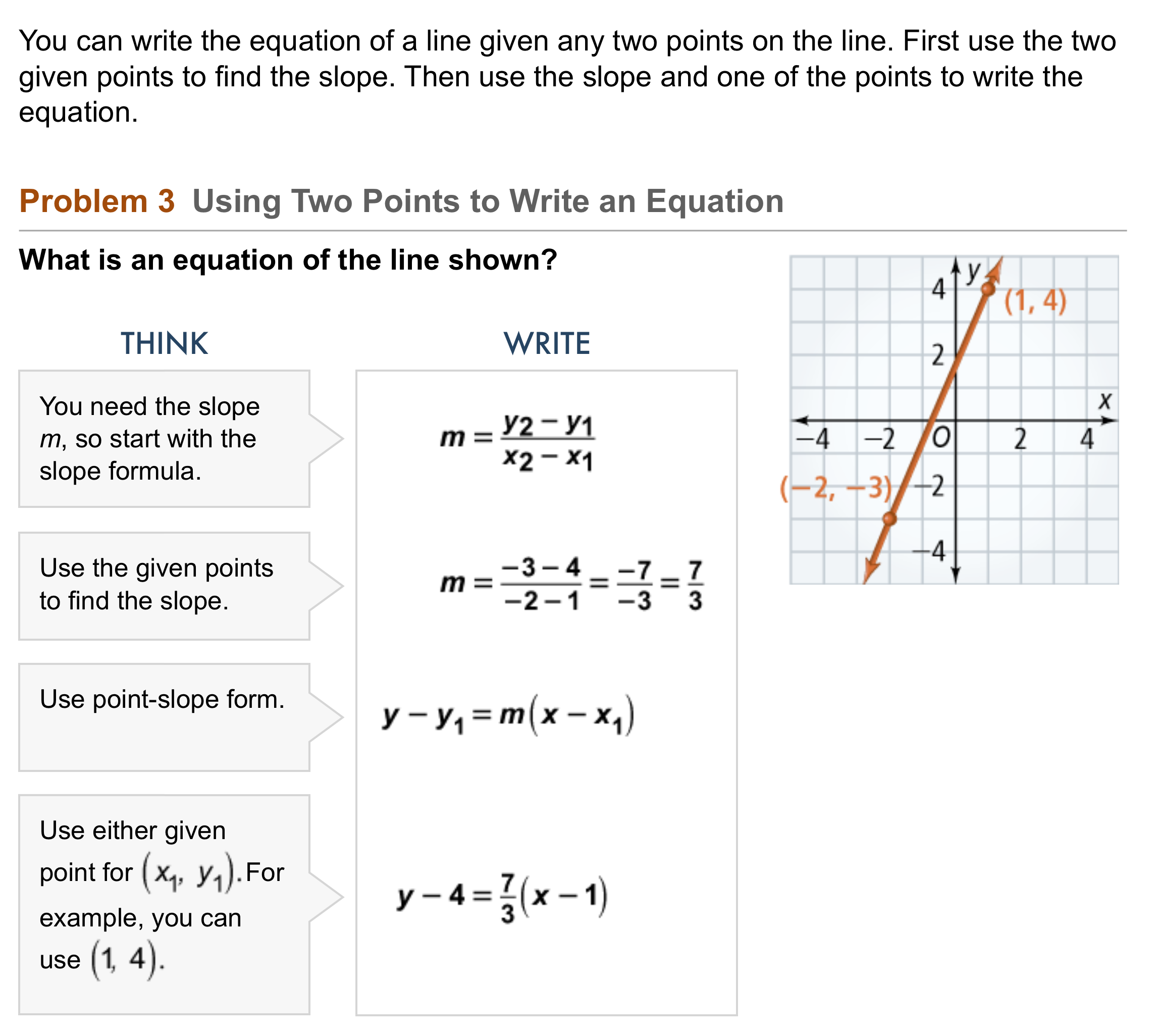Algebra 1 5-4 Complete Lesson: Point-Slope Form
starstarstarstarstarstarstarstarstarstar
5 (1 rating)
by Matthew Richardson
| 24 Questions
Note from the author:
A complete formative lesson with embedded slideshow, mini lecture screencasts, checks for understanding, practice items, mixed review, and reflection. I create these assignments to supplement each lesson of Pearson's Common Core Edition Algebra 1, Algebra 2, and Geometry courses. See also mathquest.net and twitter.com/mathquestEDU.The outlined content above was added from outside of Formative.1
1
10 pts
Solve It! The red line shows the altitude of a hot-air balloon during its linear descent. What is an equation of the line in slope-intercept form? Hint: What is the altitude of the balloon when it starts its descent at x = 0?
y = 2x + 650
y = -10x + 640
y = -2x + 6502
2
10 pts
Problem 1 Got It?
A
B
C
D
3
10 pts
Problem 2 Got It? What is the graph of the equation?
Be sure to include relevant graph detail: label axes, indicate units on both axes, and use arrows to represent end behavior, as appropriate.4
5
4
10 pts
Problem 3 Got It? In the last step of Problem 3, use the point (-2, -3) instead of (1, 4) to write an equation of the line.
y - 4 = (7/3)(x - 2)
y + 3 = (7/3)(x + 2)
y - 3 = (7/3)(x - 2)
y - 4 = (7/3)(x + 2)
5
10 pts
Problem 3 Got It? Reasoning: Rewrite the equations in Problem 3 and in the previous item in slope-intercept form. Compare the two rewritten equations. What can you conclude?6
7
6
20 pts
Problem 4 Got It? The table shows the number of gallons of water y in a tank after x hours. The relationship is linear. What is an equation in point-slope form that models the data? What does the slope represent?
7
10 pts
Problem 4 Got It? Reasoning: Rewrite the equation from the previous item in slope-intercept form. What does the y-intercept represent?8
10 pts
What are the slope and one point on the graph of the equation below?

slope: 4/9; point: (7, 12)
slope: 4/9; point: (-7, 12)
slope: 4/9; point: (-12, 7)
slope: 4/9; point: (12, 7)
9
10 pts
What is an equation of the line that passes through the point (3, -8) and has slope -2?
y + 8 = -2(x + 3)
y - 8 = -2(x + 3)
y + 8 = -2(x - 3)
y - 8 = -2(x - 3)
10
10 pts
What is an equation of the line that passes through the points (-1, -2) and (2, 4)?
y + 2 = 2(x - 1)
y + 1 = 2(x - 2)
y + 1 = 2(x + 2)
y + 2 = 2(x + 1)
11
10 pts
What is the graph of the equation below?
Be sure to include relevant graph detail: label axes, indicate units on both axes, and use arrows to represent end behavior, as appropriate.12
10 pts
Vocabulary: What features of the graph of the equation below can you identify?

13
10 pts
Reasoning: Does the graph of the equation below include the point (-2, 1)? Explain.

14
10 pts
Reasoning: Can any equation in point-slope form also be written in slope-intercept form? Give an example to explain.15
10 pts
Review Lesson 5-3: Match the slopes m and y-intercepts b with their equations.
• m = 1
• m = -1
• m = 6
• b = 0
• b = 4
• b = -13
• y = x + 4
• y = 6x
• y = -x - 13
16
10 pts
Review Lesson 2-5: Solve the equation for y.

y = 7xz
y = z/7x
y = 7z/x
17
10 pts
Review Lesson 2-5: Solve the equation for y.

y = (7b - 3) / a
y = (3b + 7) / a
y = (7b + 3) / a
18
10 pts
Review Lesson 2-5: Solve the equation for y.

y = c/6 - x
y = 6c - x
y = -c/6 + x19
10 pts
Vocabulary Review: Classify each equation based on its y-intercept.
• y = 3x + 4
• y = 4x - 3
• y = 5x + 3
• y = -3x + 2
• Has a y-intercept of 3
• Has a y-intercept other than 3
20
10 pts
Vocabulary Review: Categorize each equation based on whether or not it is in slope-intercept form.
• 2x - y = 10
• x + 3y + 11 = 0
• y - 4 = 2(x + 7)
• y = 2x + 6
• Slope-intercept form
• NOT Slope-intercept form
21
10 pts
Vocabulary Review: Classify each statement based on whether or not it is true about the y-intercept of any graph.
• occurs where y = 0 on the graph
• occurs where x = 0 on the graph
• occurs where the graph touches the x-axis
• True of the y-intercept of any graph
• NOT true of the y-intercept (of at least some graphs)22
10 pts
Use Your Vocabulary: Complete each sentence with the appropriate word from the list.
• price
• sun
• time
• The length of a shadow is a function of the angle of the __?__.
• The amount of water that has leaked from a leaky faucet is a function of __?__.
• The amount of sales tax you pay is a function of the item's __?__.
23
100 pts
Notes: Take a clear picture or screenshot of your Cornell notes for this lesson. Upload it to the canvas. Zoom and pan as needed.

For a refresher on the Cornell note-taking system, click here.
24
10 pts
Reflection: Math Success
Add to my formatives list

Formative uses cookies to allow us to better understand how the site is used. By continuing to use this site, you consent to the Terms of Service and Privacy Policy.